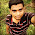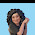c program to find sum of even number

c program to find sum of even N number

Program:-
Method 1
c program to find sum of even number using for loop

Method 2
c program to find sum of even number using while loop

Method 3
c program to find sum of even number using do while loop

Output:-
Enter a number
6
Sum of 6 even number is 12

1.thanx for ur program

1.Welcome dude :)

2.this is not true!

3.Thanks a lot for sharing this program. I found one more C Program to Find Sum of All Even Numbers.

4.wow its working thanks buddy

5.can any write a program to print the product of even numbers from 1 to 20 using while loop....

6.how to add an negative integer with this